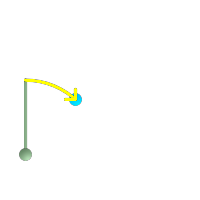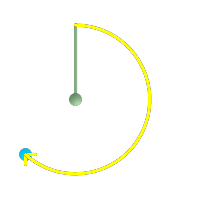## Name

ST_Azimuth — Returns the north-based azimuth of a line between two points.

## Synopsis

`float ST_Azimuth(`geometry pointA, geometry pointB`)`;

`float ST_Azimuth(`geography pointA, geography pointB`)`;

## Description

Returns the azimuth in radians of the line segment defined by the given point geometries, or NULL if the two points are coincident. The azimuth angle is referenced from north (the positive Y axis), and is positive clockwise: North = 0; Northeast = π/4; East = π/2; Southeast = 3π/4; South = π; Southwest 5π/4; West = 3π/2; Northwest = 7π/4.

For the geography type, the azimuth solution is known as the inverse geodesic problem.

The azimuth is a mathematical concept defined as the angle between a reference plane and a point, with angular units in radians. The result value in radians can be converted to degrees using the PostgreSQL function `degrees()`.

Azimuth can be used in conjunction with ST_Translate to shift an object along its perpendicular axis. See the `upgis_lineshift()` function in the PostGIS wiki for an implementation of this.

Availability: 1.1.0

Enhanced: 2.0.0 support for geography was introduced.

Enhanced: 2.2.0 measurement on spheroid performed with GeographicLib for improved accuracy and robustness. Requires PROJ >= 4.9.0 to take advantage of the new feature.

## Examples

Geometry Azimuth in degrees

```SELECT degrees(ST_Azimuth( ST_Point(25, 45),  ST_Point(75, 100))) AS degA_B,
degrees(ST_Azimuth( ST_Point(75, 100), ST_Point(25, 45) )) AS degB_A;

dega_b       |     degb_a
------------------+------------------
42.2736890060937 | 222.273689006094
```Green: the start Point(25,45) with its vertical. Yellow: degA_B as the path to travel (azimuth).Green: the start Point(75,100) with its vertical. Yellow: degB_A as the path to travel (azimuth).